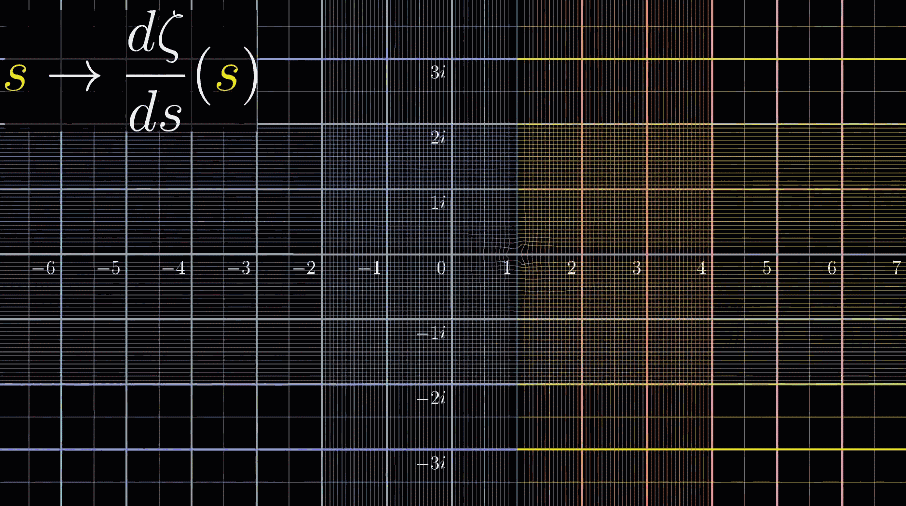【阅读时间】15min - 20min | 9000+字 | 23张动图 | 11张图片
【阅读内容】一文搞懂什么是黎曼猜想。在复数域直观可视化黎曼$\zeta$函数（读作/zita/），解释什么是解析延拓（analytic continuation），探究黎曼猜想和素数的关系

【说明】本文科普为主，是3B1B视频（版权来源）的笔记。会有很多废话来增加信息冗余度，方便对复分析之类概念不熟悉的读者理解。有基础的读者可以选择跳过一些在你们看来是在说废话的内容。就像重要的事情说三遍一样，废话增加信息的冗余度，减慢节奏，降低认知负担，希望这么做后能有更多人真正看懂什么是黎曼猜想

# 八卦黎曼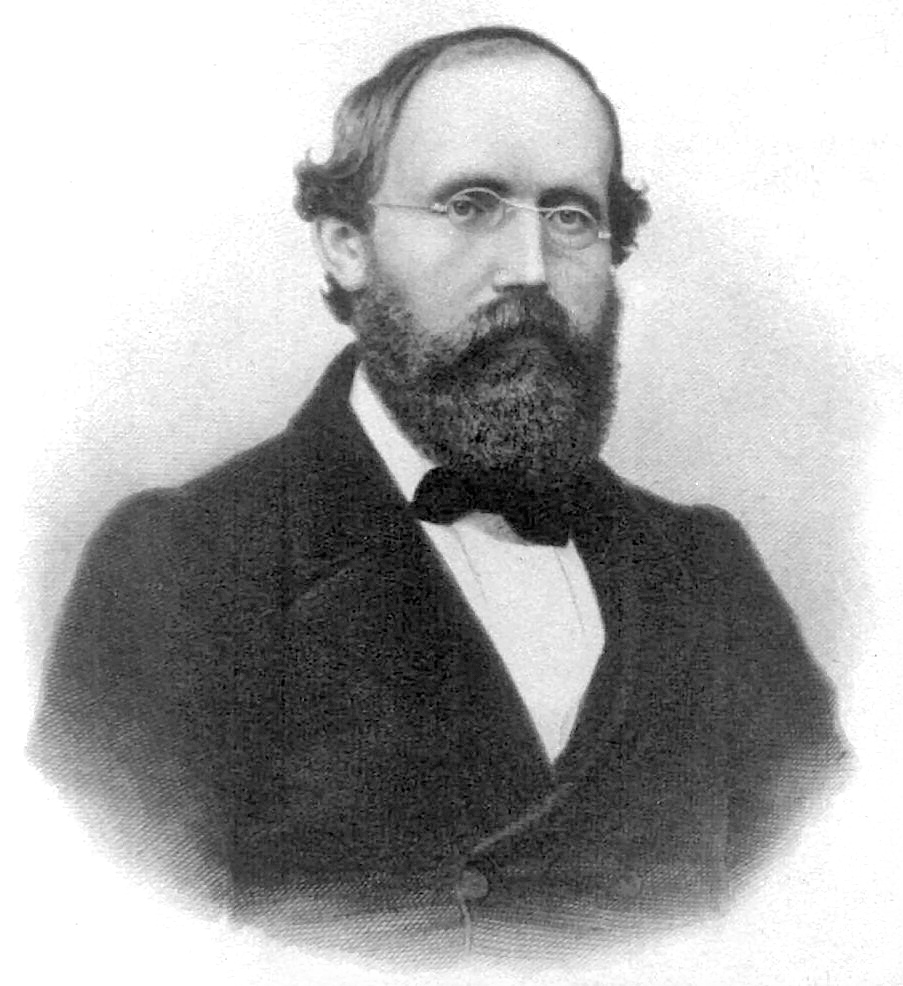## 历史背景

1806年拿破仑覆灭统治几百年的神圣罗马帝国。1815年以日耳曼民族为主的德意志邦联成立，在这个联邦中，以普鲁士奥地利最为强大，两大势力得争个高下。一直到1862年脾斯麦执政，才走向一统。最终，1871年，德意志帝国成立，黎曼没能见到这一天

19世纪5、60年代普鲁士完成了工业革命，这必定伴随着思想和科学的蓬勃发展。黎曼是生在一个好时代，这个时代，整个科学领域，一片蓝海，名家辈出，群星璀璨

## 成就

【补充1】因为代数几何中有关椭圆曲线的相关研究还没有兴盛，著名的费马大定理：$x^n + y^n = z^n \; 当\;n>2\;没有整数解$ 未出现在列表中，虽然当时这个猜想也没有被证明

【补充2】在希尔伯特的问题列表中，黎曼猜想并不单独为一题。包含黎曼猜想的第8题是：黎曼猜想哥德巴赫猜想孪生素数猜想，这每一个猜想都闻名遐迩。三位一体，由此可见这三个问题之间是存在关联的

21世纪黎曼猜想又被列为千禧年7道世纪难题之一，克雷数学研究所承诺：解决一道题 ➜ 100万美元

P/NP问题 | 霍奇猜想 | 庞加莱猜想（已证明）| 黎曼猜想 | 杨-米尔斯存在性与质量间隙 | 纳维-斯托克斯存在性与光滑性 | 贝赫和斯维讷通-戴尔猜想

# 黎曼猜想的专业定义

【补充（只为严谨，可以跳过）】把上面的式子称为黎曼$\zeta$函数并不严谨，严谨的来说：定义域必须纳入考虑，才能完整写出黎曼$\zeta$函数的形式

$$\zeta(s) = \sum_{n=1}^{\infty} \frac{1}{n^s}$$

$$\zeta(s) = \frac{1}{\Gamma(s)}\int_{0}^{\infty} \frac{x^{s-1}}{e^x - 1} dx$$

• ❓ 黎曼$\zeta$函数是个什么函数若读者的数学基础为高中，那么博主猜测您有疑惑的是…这个符号，它的含义很简单：按照1 2 3 4每次加1的规律一直重复前一项的形式。举个例子：

$$\zeta(s) = \frac{1}{1^s} + \frac{1}{2^s} + \frac{1}{3^s} + \frac{1}{4^s} + \frac{1}{5^s} + \frac{1}{6^s} + \frac{1}{7^s} + \frac{1}{8^s} + \cdots = \sum_{n=1}^{\infty} \frac{1}{n^s}$$

• ❓ 什么是非平凡零点本博文想说清楚的问题
• 实数部分指的什么？难不成还有虚数部分？ 这部分涉及虚数的概念，虚数定义为 $\sqrt{-1}$ 记为符号 i，接下来就需要一些复数基础，这一点跳不过，但本博文也尝试帮你解决这个问题
• ❓ $\frac{1}{2}$ 这个数是怎么来的？ 本博文想说清楚的问题

# 可视化黎曼$\zeta$函数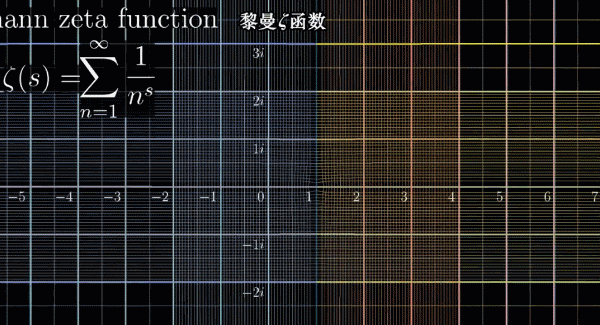## 黎曼$\zeta$函数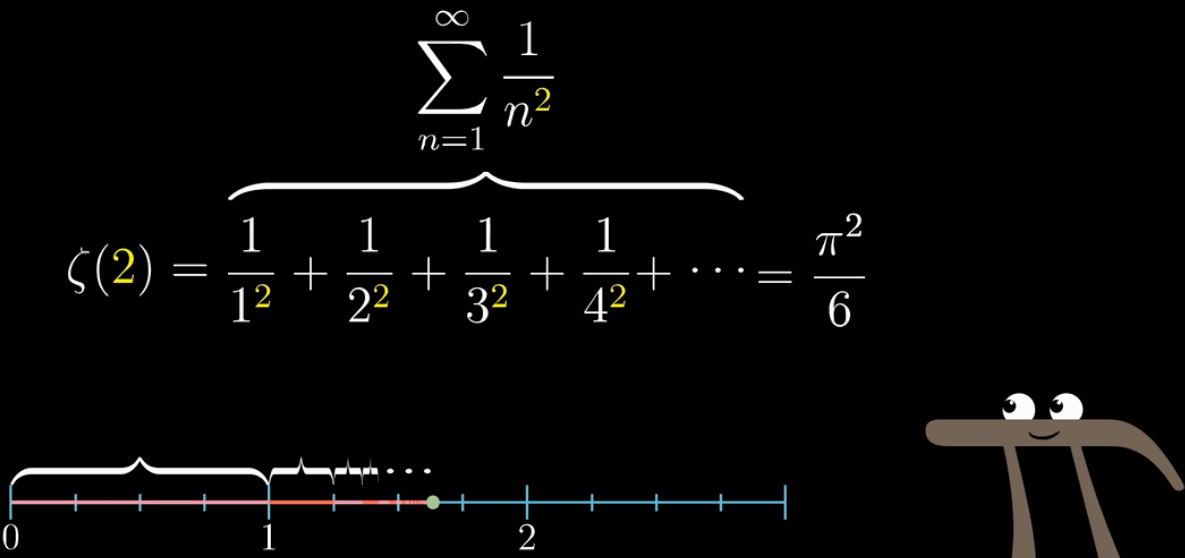## 定义域扩展到复数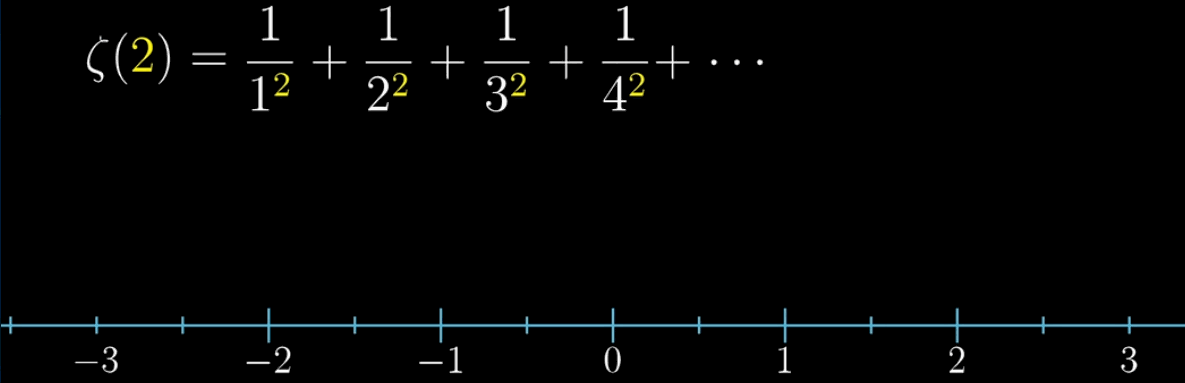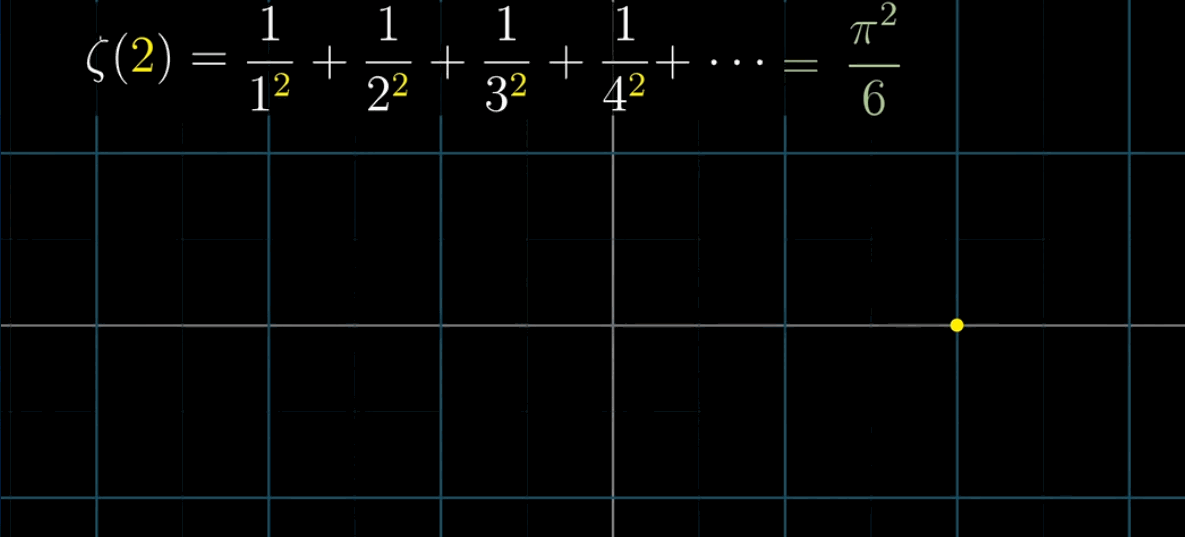❓这里可能会出现两个问题 ➜ ① 什么是复平面？ ② $\left(\cfrac{1}{2}\right)^{2+i}$ 怎么计算？几何含义是什么？

### 复平面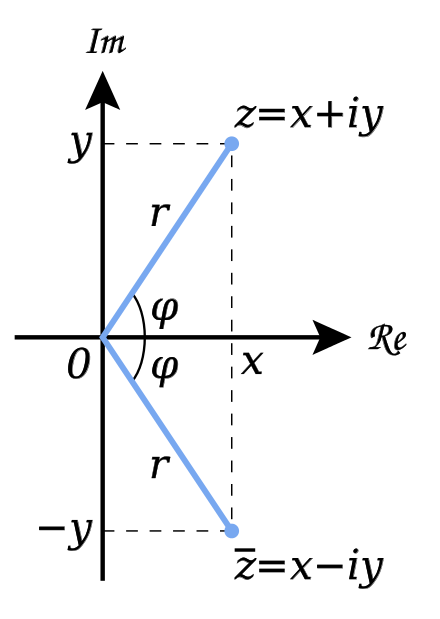### 虚数单位为幂指数

$2^x$ ，这个 $x$ 就是幂指数，$2^i$ 即虚数单位为幂指数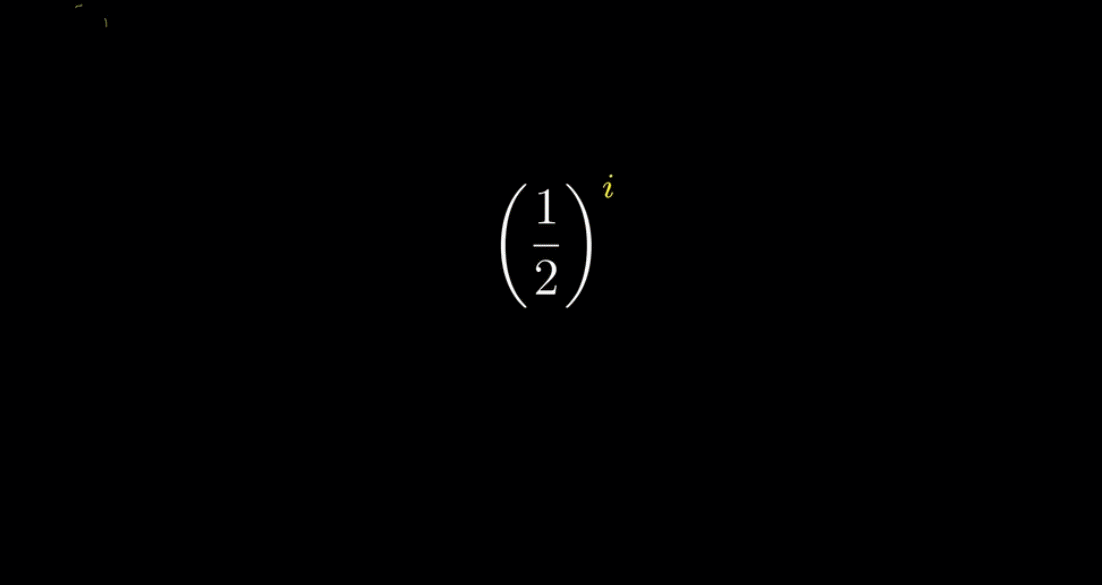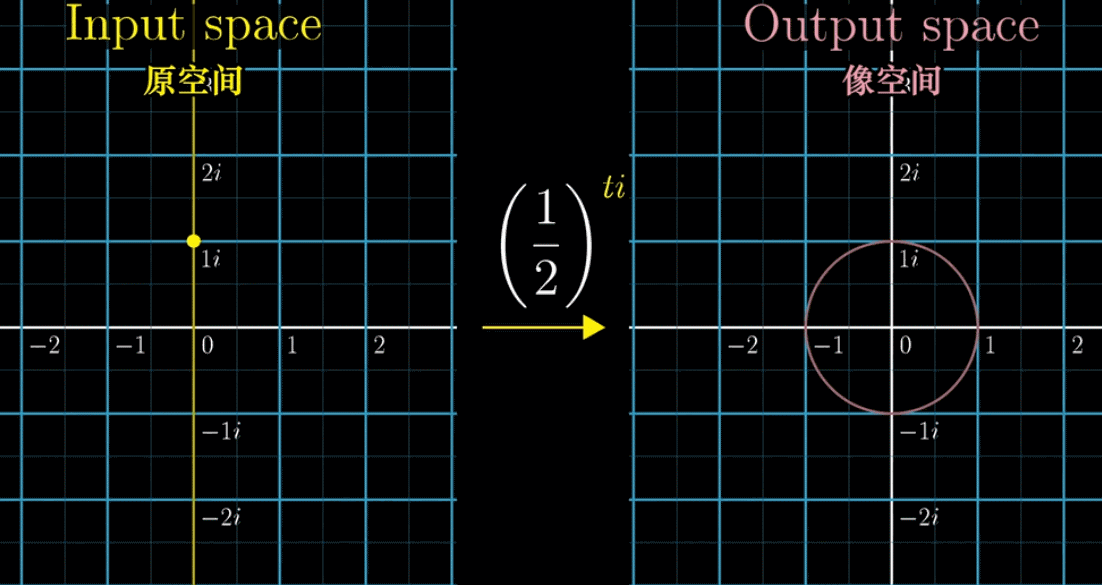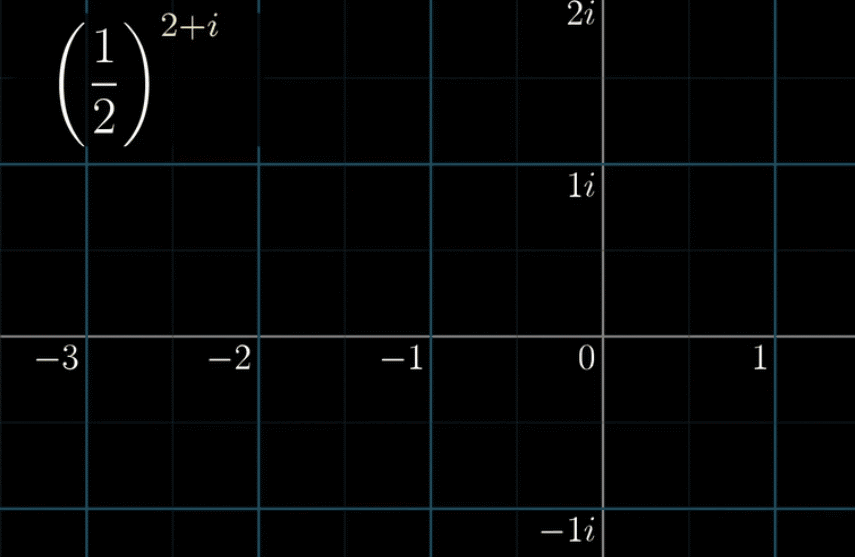① 实部把点收缩到 $\cfrac{1}{4}$ 的位置

② $\left( \cfrac{1}{2}\right)^i$ 不改变长度（因为是虚部），只旋转一个对应的角度

## ⭐️一步一步可视化

### 进行旋转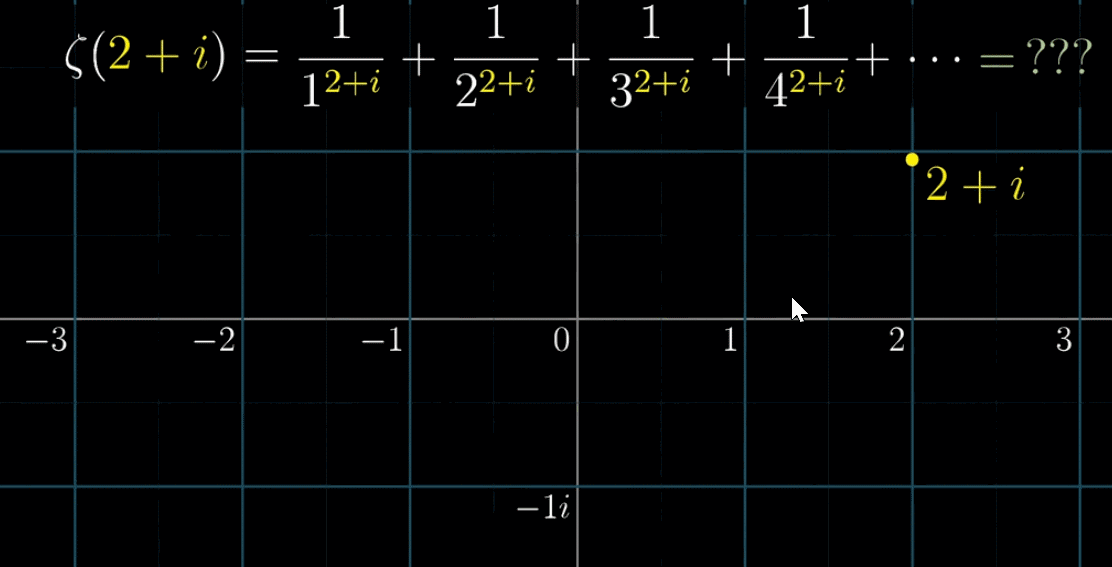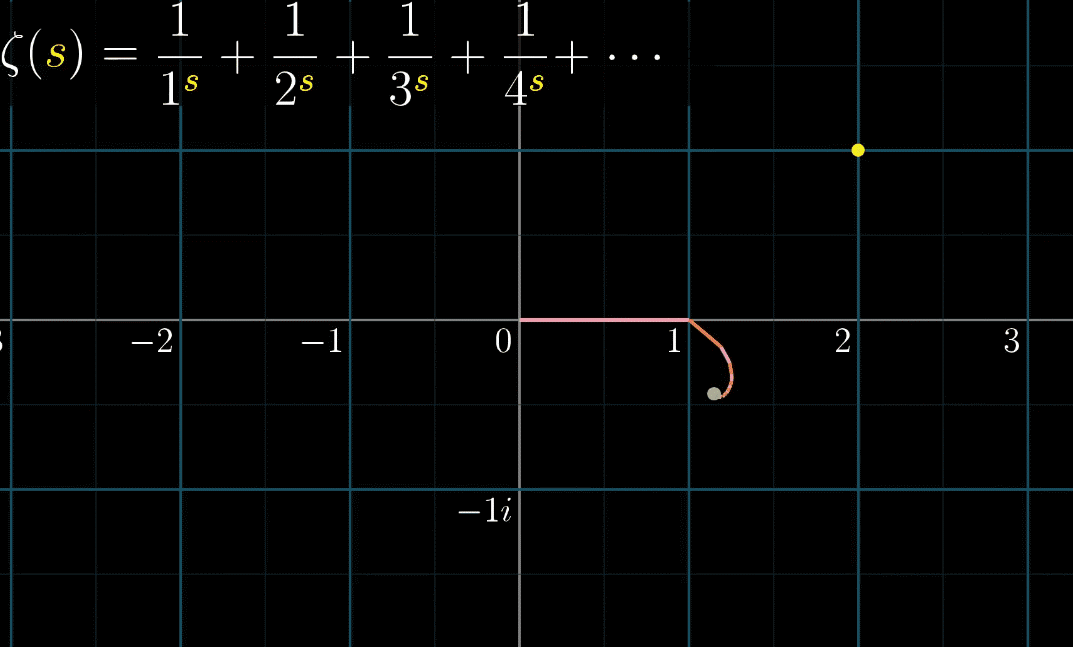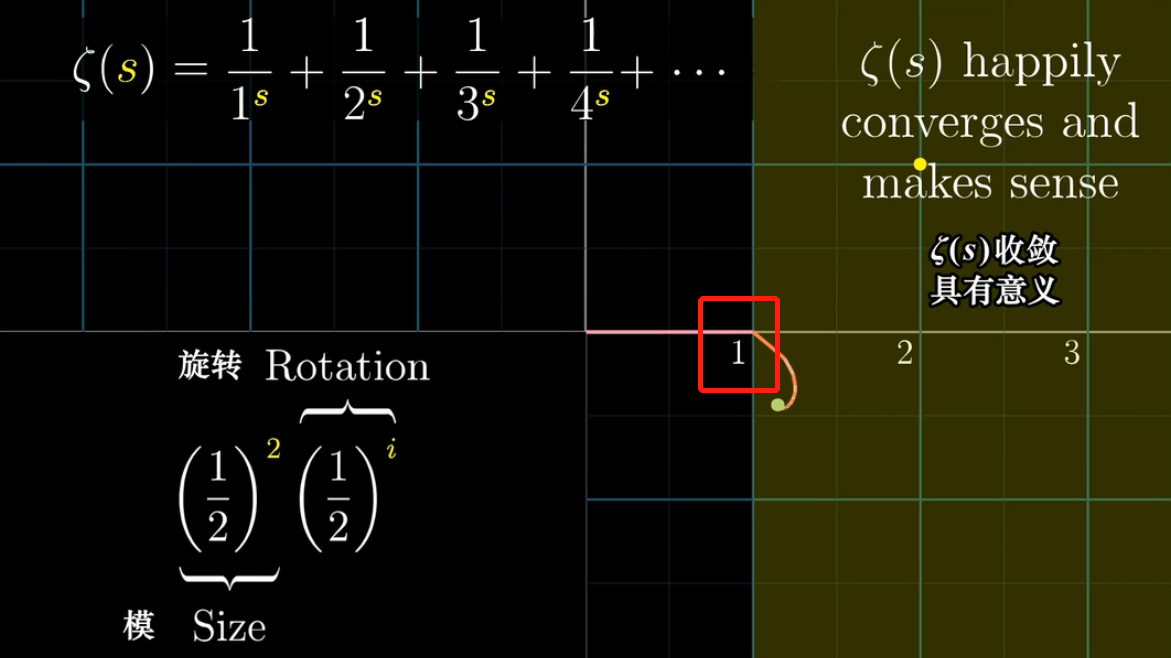### 变换黎曼$\zeta$函数

$$f(s) = s^2$$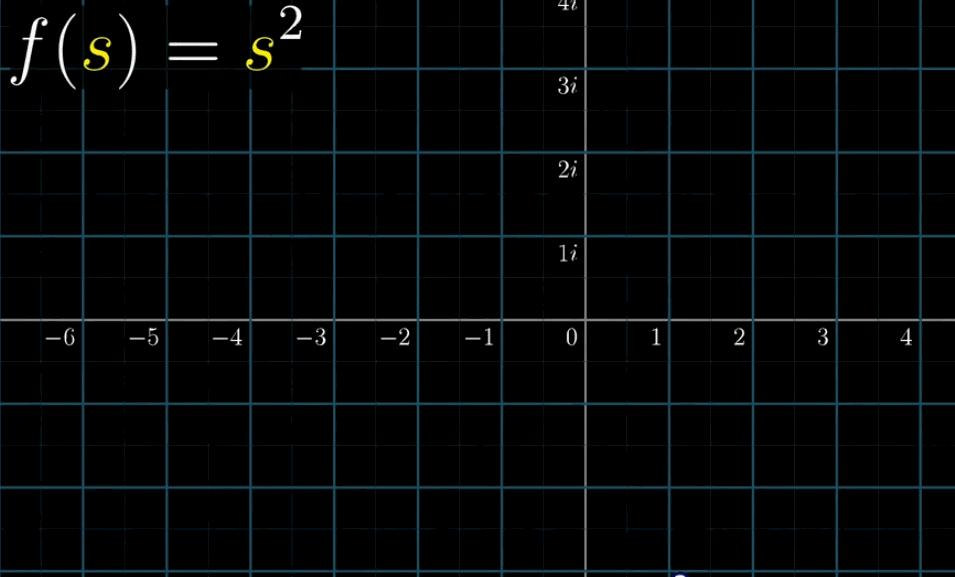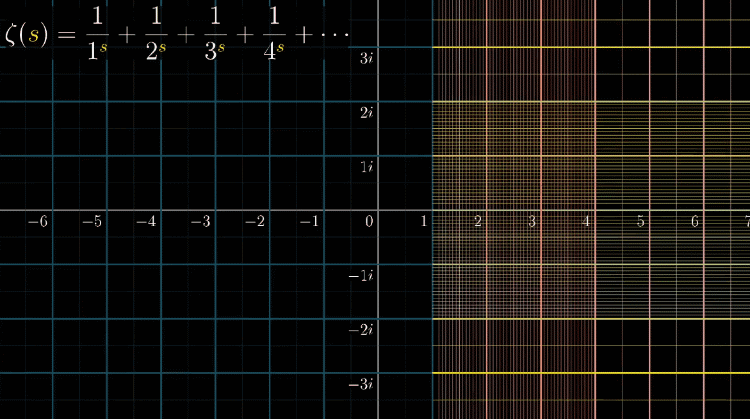## 难受的停顿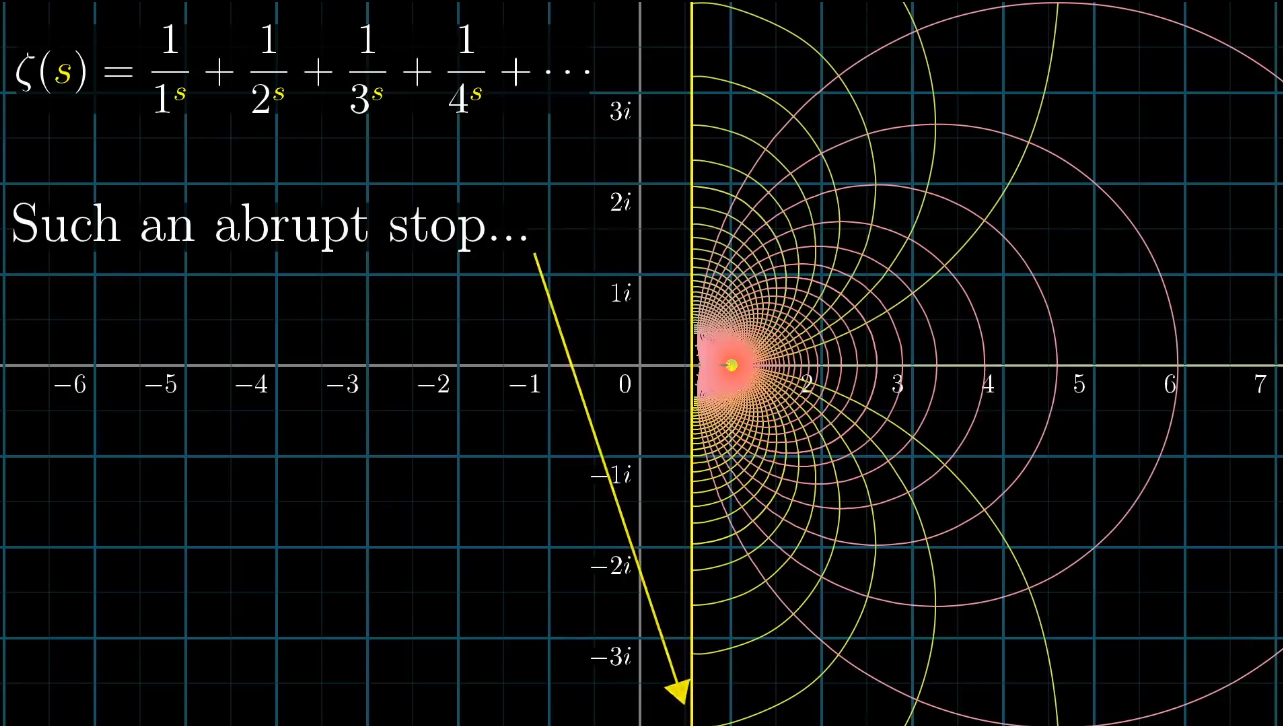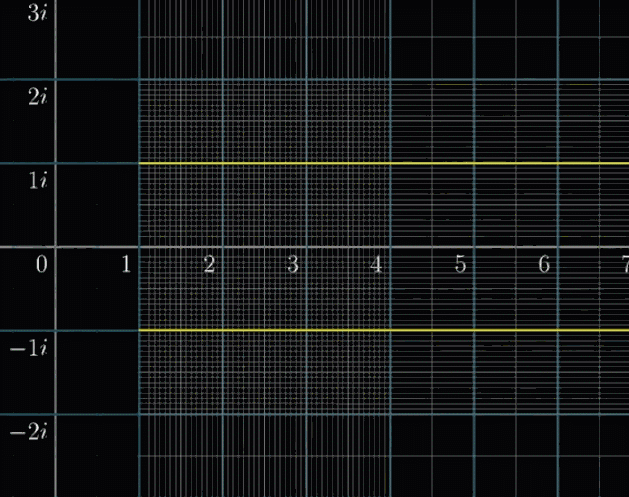## 解析延拓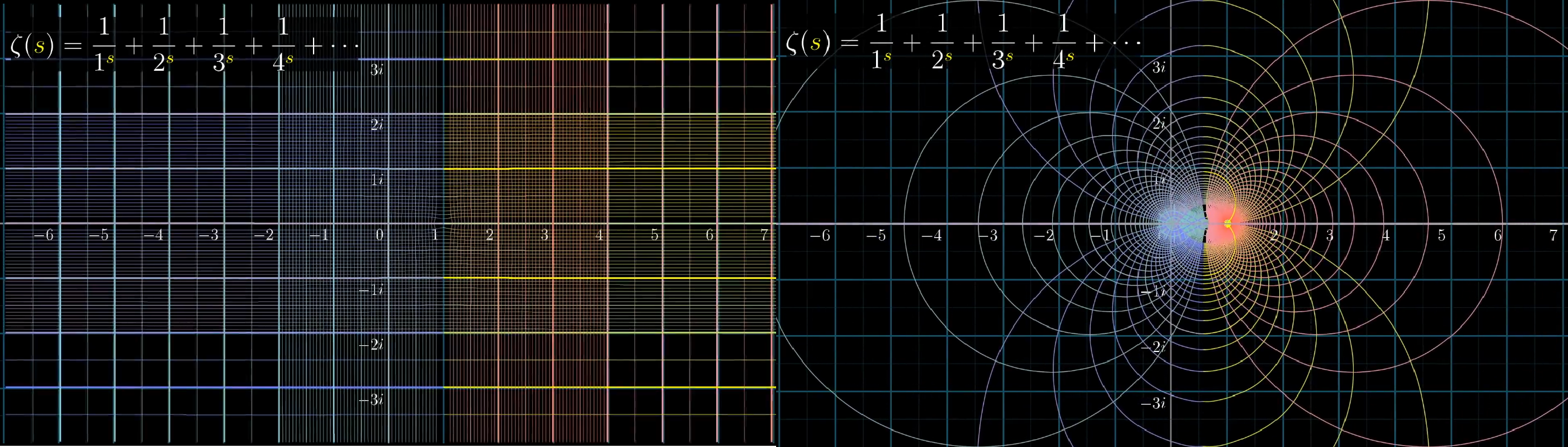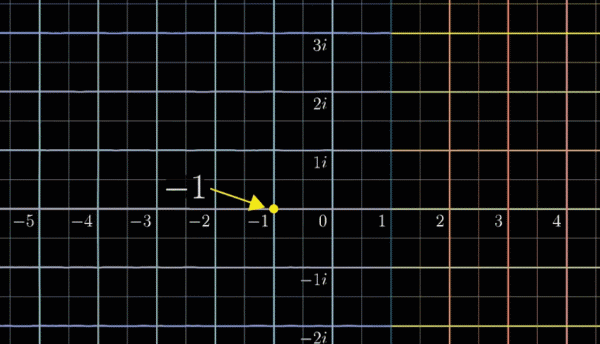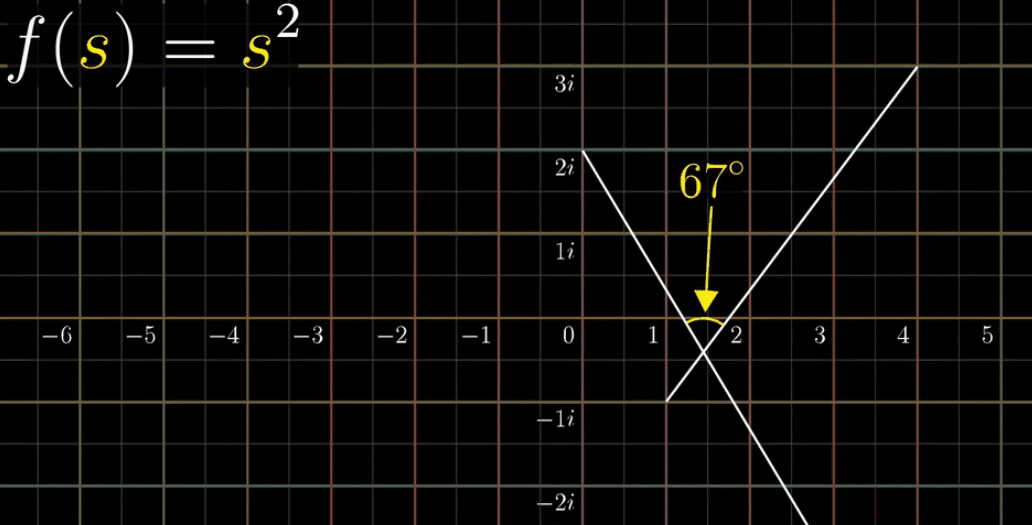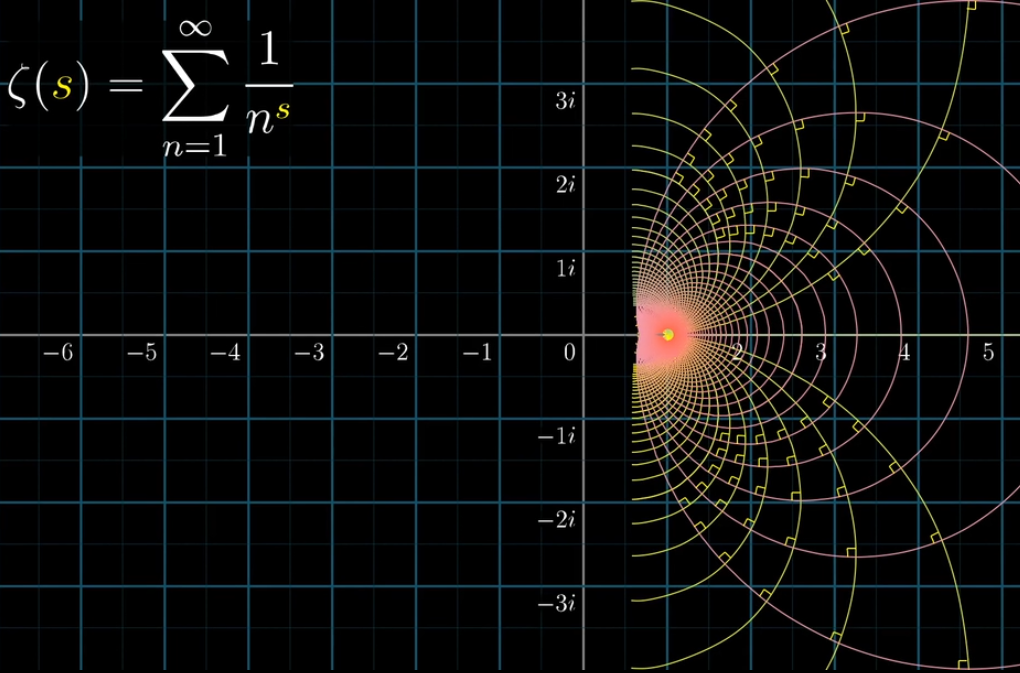## 可视化黎曼$\zeta$函数总结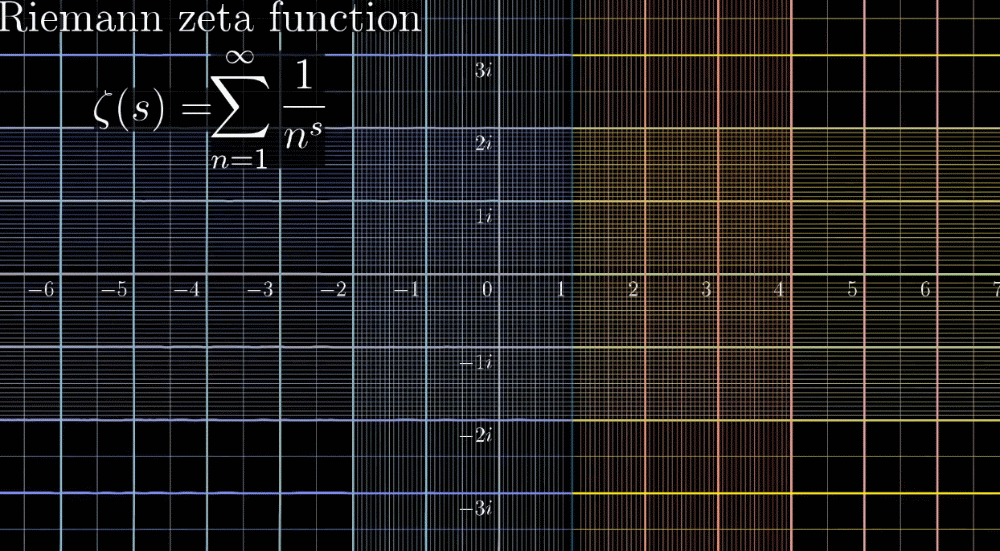$$\zeta(s) = 2^s{\pi}^{s-1}\sin(\frac{\pi s}{2})\Gamma(1-s)\zeta(1-s)$$

# 素数规律和黎曼猜想

## 再看黎曼猜想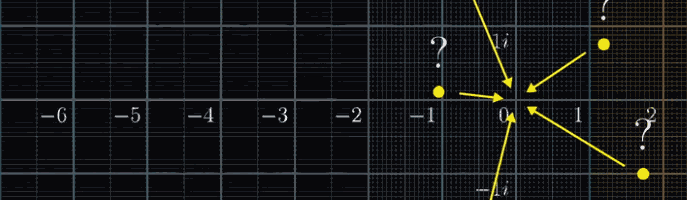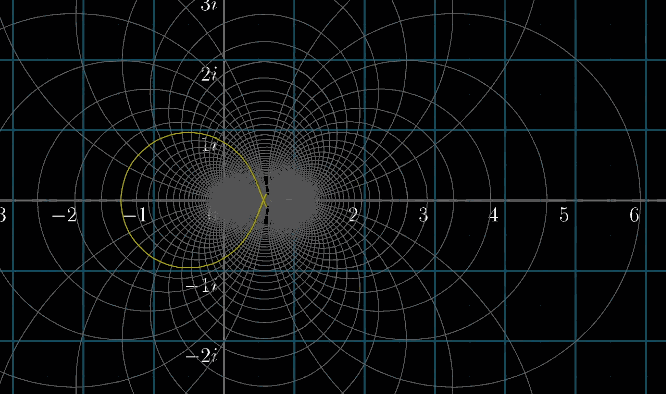## 素数规律

### 素数个数

$$q = 2×3×5×\cdots × p + 1 \tag 1$$
① 假设 $q$ 是质数 ➜ $q\gt p$ 这和 $p$ 是最大的质数这个假设矛盾

② 假设 $q$ 是合数（不是质数的数） ➜ $q$ 是有约数的，不是1也不是它本身 ➜ 那就一定是(1)式中 $2×3×5×\cdots × p$ 中的某一个，但是由（1）式可得，$q$ 除以$2×3×5×\cdots × p$ 中任何一个数都余1 ➜ 所以肯定不能整除与假设 $q$ 是合数矛盾

### 欧拉乘积公式

$$\prod\limits_{p} (1-p^{-s})^{-1} = \frac{1}{1-\cfrac{1}{2^s}}×\frac{1}{1-\cfrac{1}{3^s}}×\frac{1}{1-\cfrac{1}{5^s}}×\cdots = \cfrac{1}{1^s}+\cfrac{1}{2^s}+\cfrac{1}{3^s}+\cdots$$

### 素数定理$$\pi(x) \sim \frac{x}{\ln x} \iff \pi(x) = \int_{0}^{x} \frac{dt}{\ln t} + C$$
$\sim$ 符号表示趋近于，也就是当 $x \to +\infty$ 的意思。 $C$ 是一个常数，这个常数随着 $x$ 的变大而越来越小

$$\pi(x) = Li（x）+ O(\sqrt{x}\ln x)$$
$O()$ 被称为渐进符号，一般用来描述无穷级数的余项。在计算和表示算法复杂度方面也很用，比如$O(n^2)$ 其实就是忽略 $n$ 一次项和常数项的意思。因为在 $n$ 非常大时， $n$ 一次项对数值的贡献在量级上远小于 $n^2$ 二次项。这个余项的常数项的具体数值还没有算出来

# 后记和思考

【参考和来源】